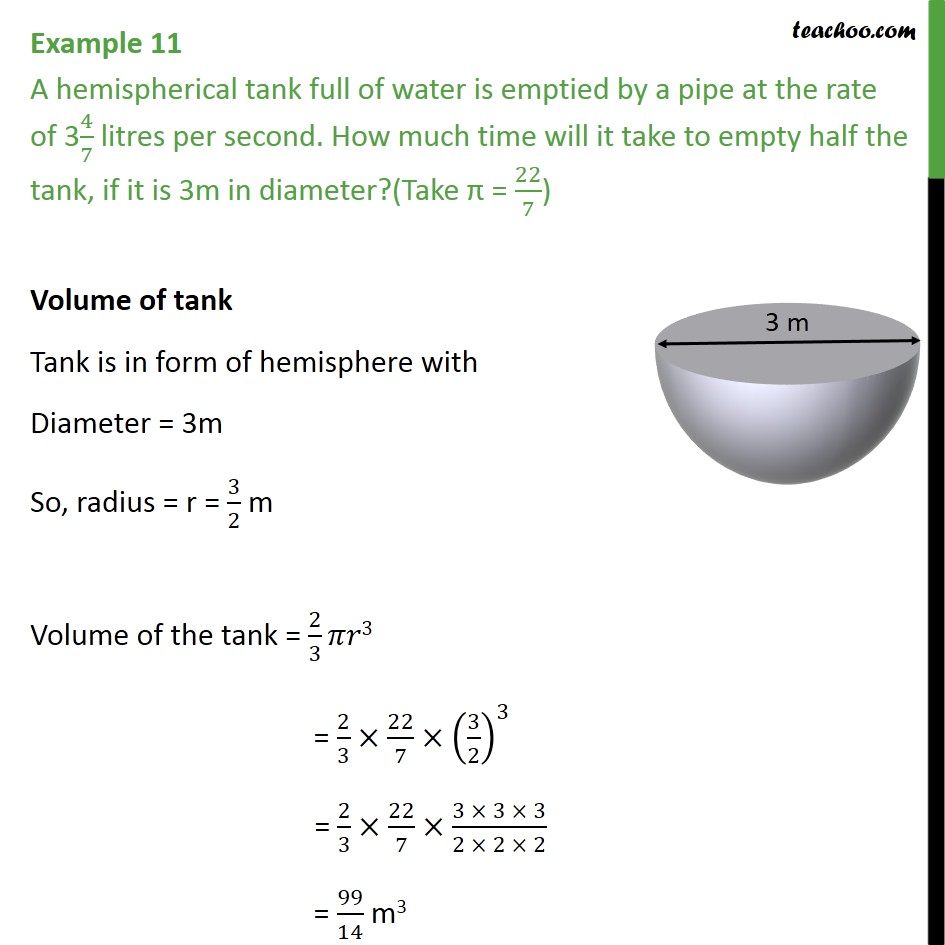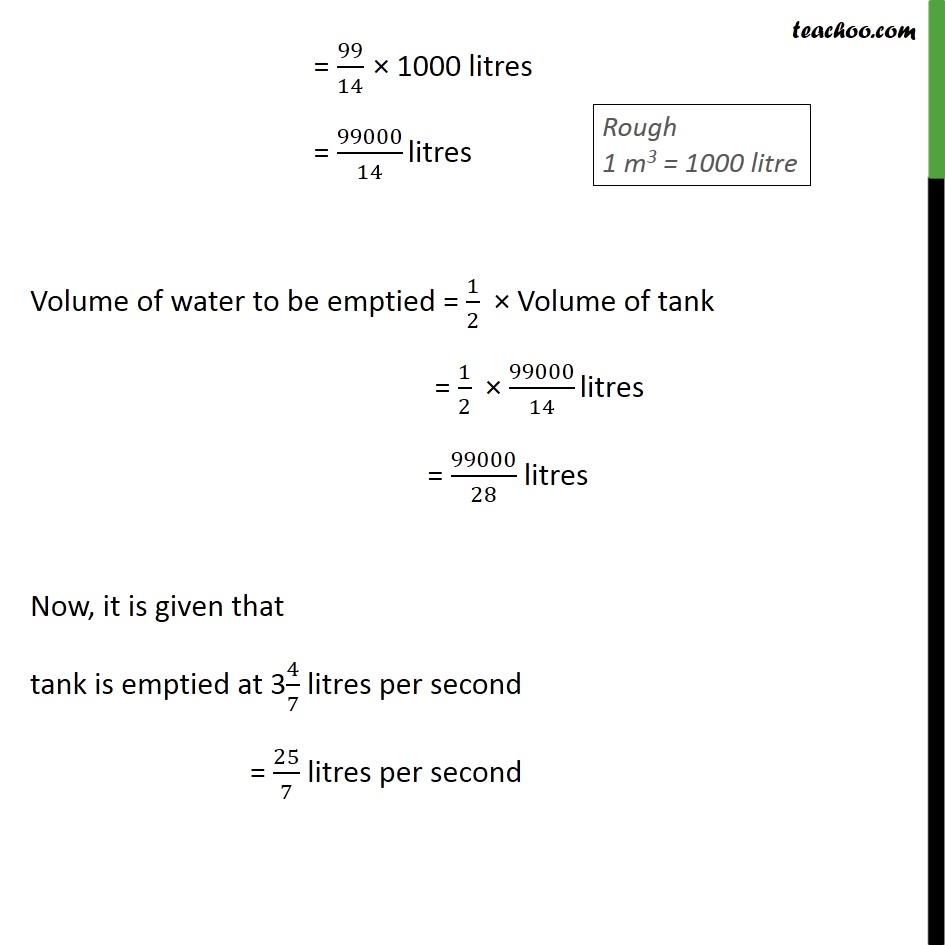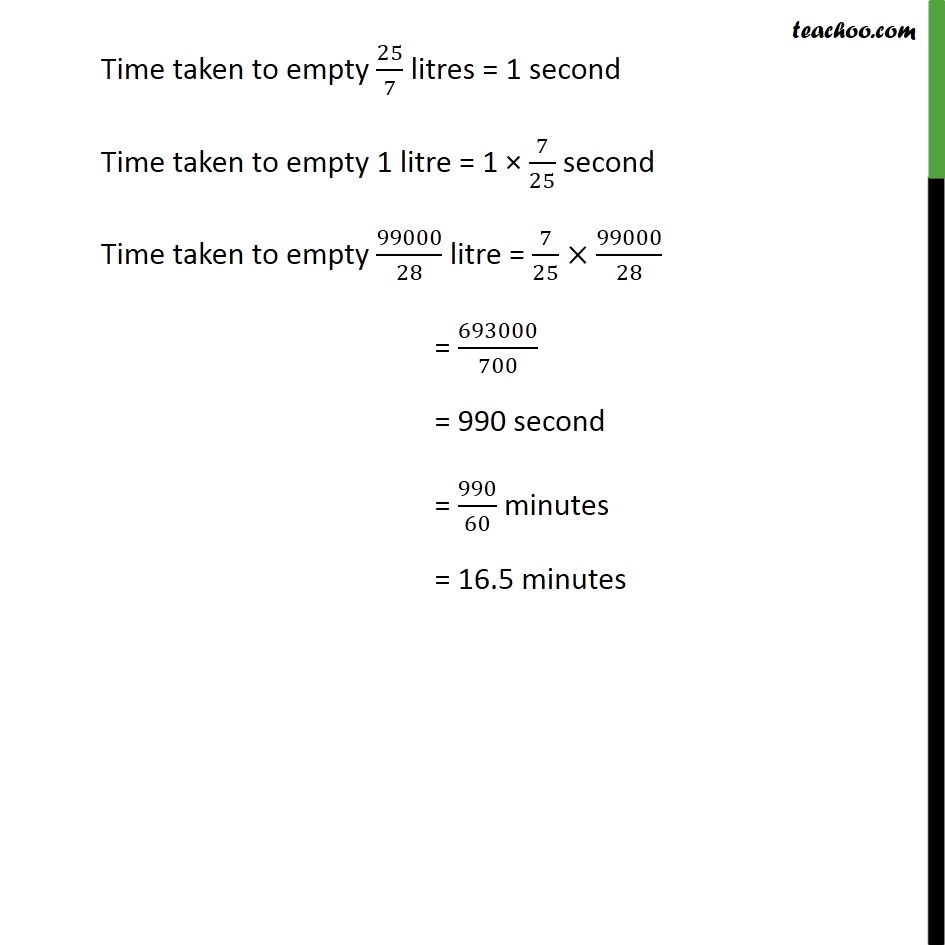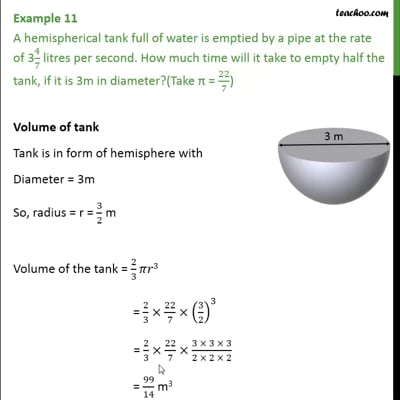Examples

Chapter 13 Class 10 Surface Areas and Volumes
Serial order wiseThis video is only available for Teachoo black users

Maths Crash Course - Live lectures + all videos + Real time Doubt solving!

### Transcript

Example 11 A hemispherical tank full of water is emptied by a pipe at the rate of 34/7 litres per second. How much time will it take to empty half the tank, if it is 3m in diameter?(Take = 22/7) Volume of tank Tank is in form of hemisphere with Diameter = 3m So, radius = r = 3/2 m Volume of the tank = 2/3 3 = 2/3 22/7 (3/2)^3 = 2/3 22/7 (3 3 3)/(2 2 2) = 99/14 m3 = 99/14 1000 litres = 99000/14 litres Volume of water to be emptied = 1/2 Volume of tank = 1/2 99000/14 litres = 99000/28 litres Now, it is given that tank is emptied at 34/7 litres per second = 25/7 litres per second Time taken to empty 25/7 litres = 1 second Time taken to empty 1 litre = 1 7/25 second Time taken to empty 99000/28 litre = 7/25 99000/28 = 693000/700 = 990 second = 990/60 minutes = 16.5 minutes